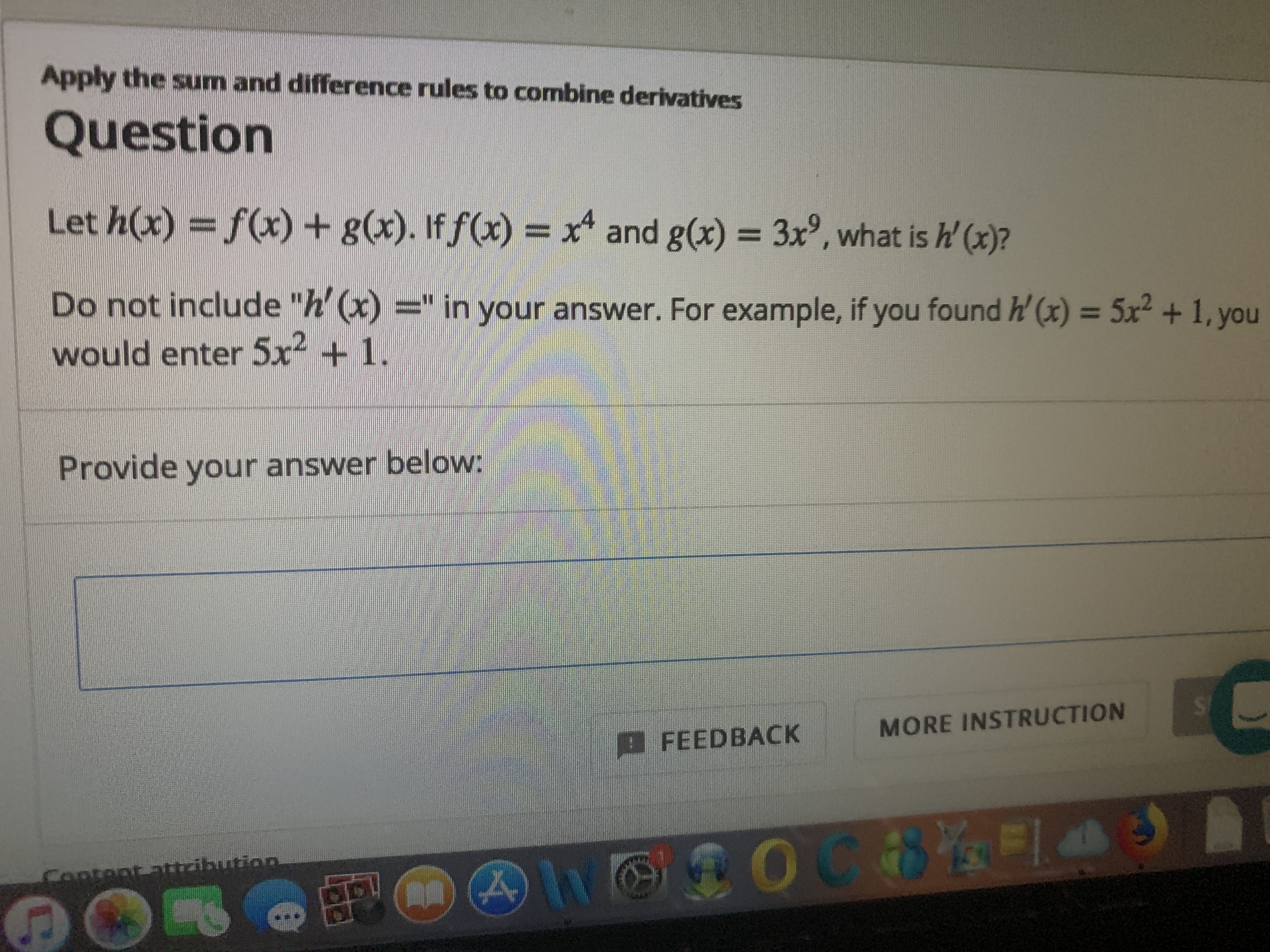# Apply the sum and difference rules to combine derivativesQuestionLet h(x) = f(x) + g(x). If f(x) = xand g(x) = 3x, what is h' (x)?Do not include "h' (x) =" in your answer. For example, if you found h' (x) = 5x + 1, youwould enter 5x2 + 1.%3DProvide your answer below:SIMORE INSTRUCTIONFEEDBACKO0Contept attribution**.

Question
28 viewshelp_outlineImage TranscriptioncloseApply the sum and difference rules to combine derivatives Question Let h(x) = f(x) + g(x). If f(x) = x and g(x) = 3x, what is h' (x)? Do not include "h' (x) =" in your answer. For example, if you found h' (x) = 5x + 1, you would enter 5x2 + 1. %3D Provide your answer below: SI MORE INSTRUCTION FEEDBACK O0 Contept attribution **. fullscreen
check_circle

Step 1

Given:

...

### Want to see the full answer?

See Solution

#### Want to see this answer and more?

Solutions are written by subject experts who are available 24/7. Questions are typically answered within 1 hour.*

See Solution
*Response times may vary by subject and question.
Tagged in

### Derivative